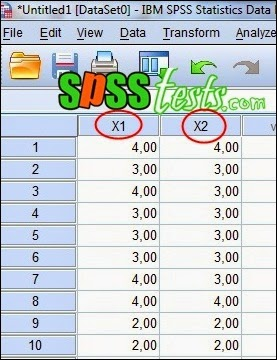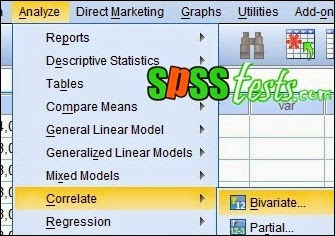# How to test Spearman Rank Correlation Coefficient Using SPSS

How to test Spearman Rank Correlation Coefficient Using SPSS | Spearman Rank Correlation Test is part of the non-parametric statistics. As it is known that the non-parametric statistic does not require the terms as contained in parametric statistics, such data must be normally distributed and have the same variant

Spearman Rank Correlation test aims to examine the relationship between two variables berdata ordinal. However, if the data in the form of a variable ratio it is more appropriate to use Correlation Pearson Product-Moment

Basic Decision Making in Spearman Rank Correlation Coefficient Test
1. If the value of Sig. <0.05, then there is a relationship between variables
2. If the value of Sig. > 0.05, there is no correlation between variables

Case in Spearman Rank Correlation Coefficient Test

A researcher wants to know whether there is a relationship between consumer satisfaction with Customer Service. Therefore, researchers collect the necessary data, so that the data collected Consumer Satisfaction and Customer Services as followsSpecification:
1 = very unsatisfied
2 = Not satisfied
3 = Satisfied
4 = Very Satisfied

Step-by-Step Test Spearman Rank Correlation Coefficient by SPSS

1. Turn on SPSS worksheet, and then click Variable View, on the Name write X1 and X2. On the Label, write Consumer Satisfaction and Consumer Services2. Next, click Data View and enter the variable values X1 and X2.3. Then, click Analyze - Correlate - bivariate ...4. The dialog box appears with the name Bivariate Correlations, then enter the variables X1 and X2 to the Variables box, on the Correlation Coefficients, uncheck Pearson, provide a check on Spearman. Tests of Significance for the column select Two-tailed, provide a check on Flaq significant correlations5. Lastly click Ok, then the output will appear as follows:Interpretation of Test Output Spearman Rank Correlation Coefficient

Based on the above known output value of Sig. (2-tailed) of 0.003 <0.05, as the basis for a decision on the above, it can be dimpulkan that there is a significant relationship between customer satisfaction with Customer Service

Next: Step by Step Simple Linear Regression Analysis Using SPSS

[Keywords: How Spearman Rank Correlation Coefficient Test Using SPSS | Case in Spearman Rank Correlation Coefficient Test | Spearman Rank Test Method using SPSS version 21 | Step-by-Step Test Spearman correlation coefficients with SPSS]

### 1 Response to "How to test Spearman Rank Correlation Coefficient Using SPSS"

1.steps in spear man method to relate to real life practicals